# Mathematica与数学–偏微分方程数值解（PDE）2017年10月5日12:02:56

5 3904字阅读13分0秒

### 知识点 -- 关于三类边界条件

1. 第一类边界是给定边界上待求变量的分布

2. 第二类边界是给定边界上待求变量的梯度值

3. 第三类边界是待求变量与梯度值之间的函数关系

### 第一题

#### 题目要求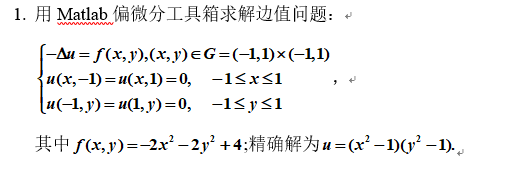#### matlab解决方法

• 设置方程的边界条件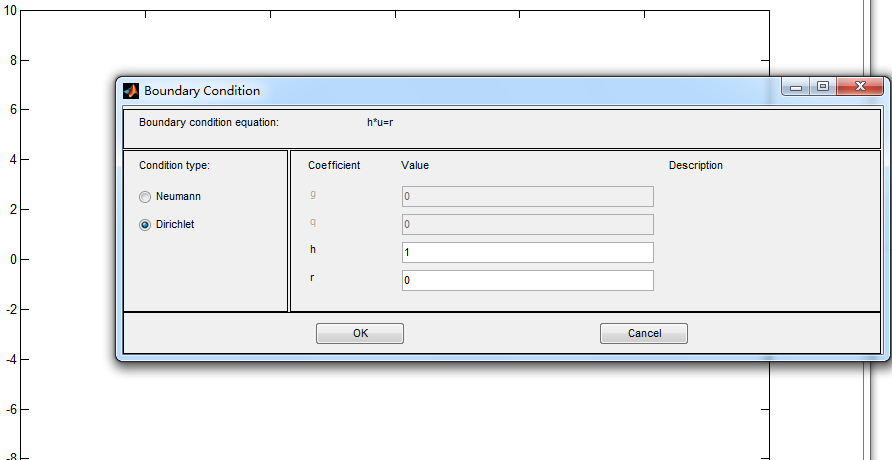• 设置要求解的方程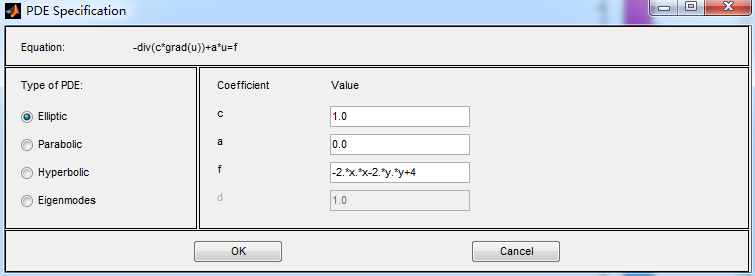• 查看解出的答案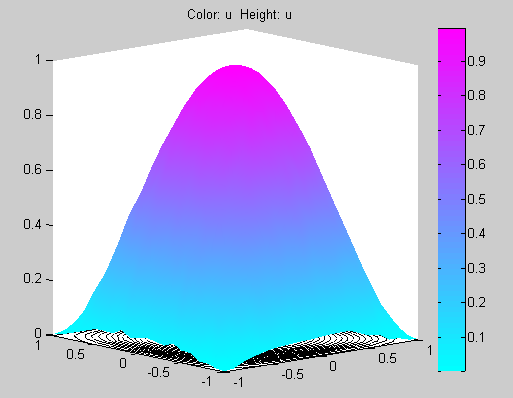#### mathematica解决方法

``````(*首先给出边界区域*)
boundaries = Rectangle[{-1, -1}, {1, 1}];
RegionPlot[boundaries]
(*利用上面区域，利用DirichletCondition来规定第一类边界*)
sol = NDSolveValue[
{-(D[u[x, y], {x, 2}] + D[u[x, y], {y, 2}]) == -2 x^2 - 2 y^2 + 4,
DirichletCondition[u[x, -1] == 0, -1 < x < 1],
DirichletCondition[u[x, 1] == 0, -1 < x < 1],
DirichletCondition[u[1, y] == 0, -1 < y < 1],
DirichletCondition[u[-1, y] == 0, -1 < y < 1]
}, u, {x, y} \[Element] boundaries]
(*绘制解出的图像*)
Plot3D[sol[x, y], {x, y} \[Element] boundaries,
ColorFunction -> "SolarColors", PlotLegends -> Automatic]``````

• 首先画出区域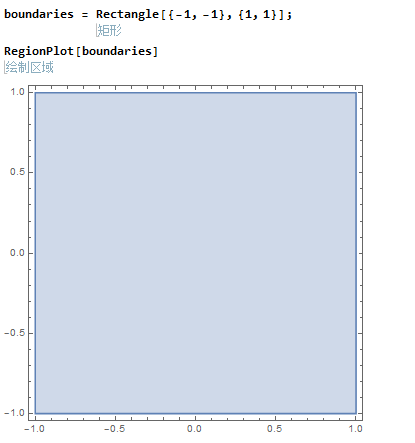• 再求解方程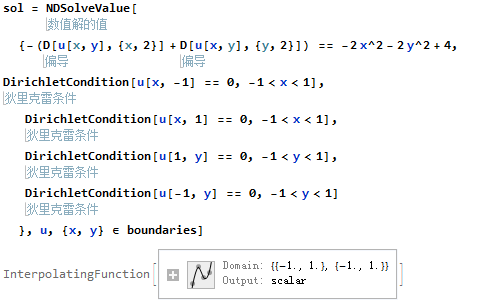• 画出图像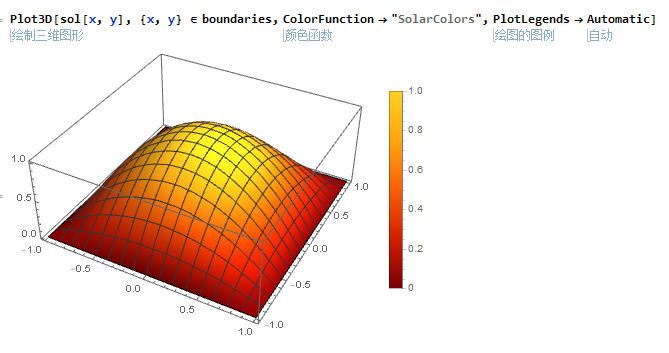#### 精确解的图像

``Plot3D[(x^2 - 1) (y^2 - 1), {x, -1, 1}, {y, -1, 1}, BoxRatios -> {1, 1, 1}]``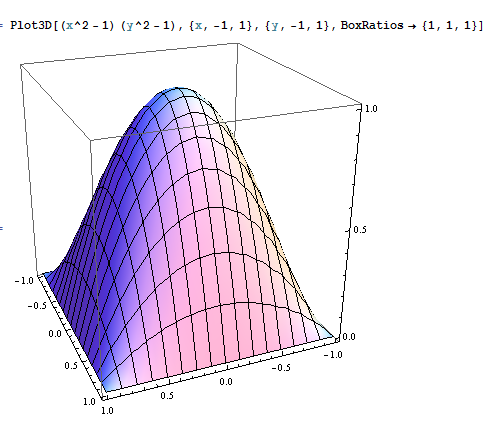### 第二题

#### 题目要求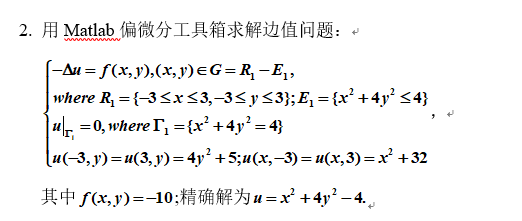#### matlab解决方法

• 设置边界条件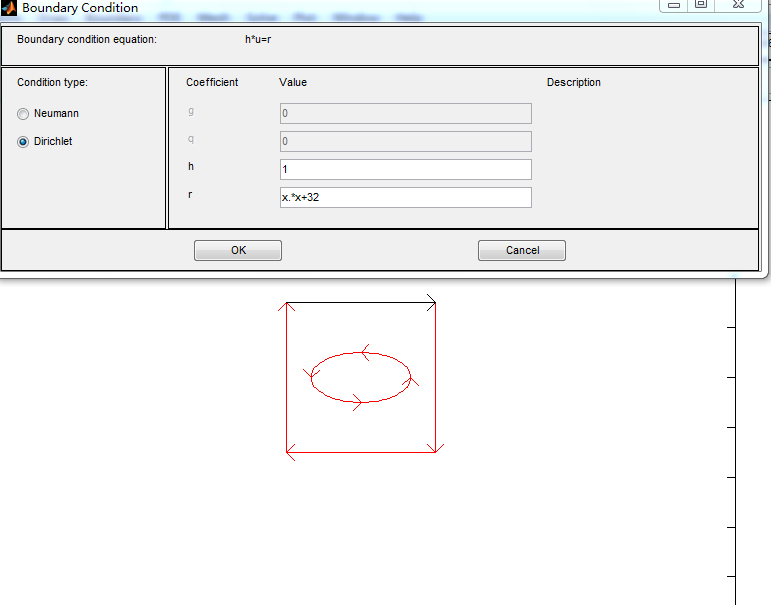• 求解结果画图显示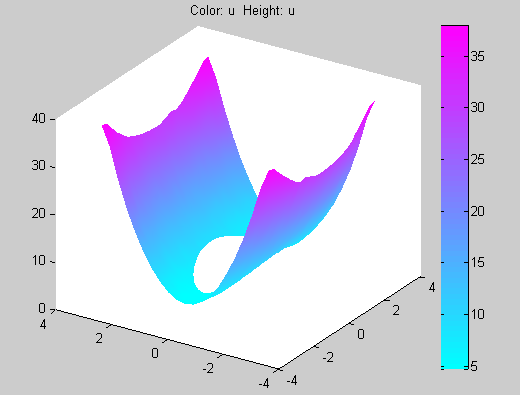#### mathematica解决方法

``````(*首先给出边界区域*)
boundaries =
ImplicitRegion[ {-3 <= x <= 3 && -3 <= y <= 3 && !x^2 + 4 y^2 < 4}, {x, y}];
RegionPlot[boundaries]
(*利用上面区域，利用DirichletCondition来规定第一类边界*)
sol = NDSolveValue[
{-(D[u[x, y], {x, 2}] + D[u[x, y], {y, 2}]) == -10,
u[-3, y] == 4*y^2 + 5,
u[3, y] == 4*y^2 + 5,
u[x, -3] == x^2 + 32,
u[x, 3] == x^2 + 32,
DirichletCondition[u[x, y] == 0, x^2 + 4*y^2 == 4] },
u, {x, y} \[Element] boundaries]
(*绘制解出的图像*)
Plot3D[sol[x, y], {x, y} \[Element] boundaries,
ColorFunction -> "SolarColors", PlotLegends -> Automatic]``````

• 首先画出区域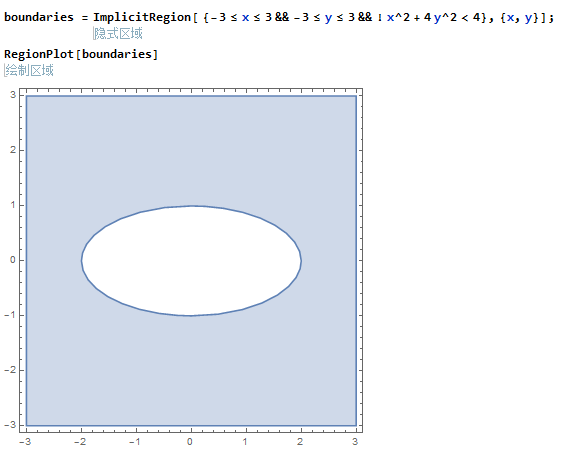• 再求解方程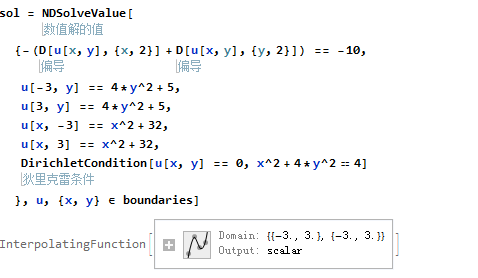• 画出图像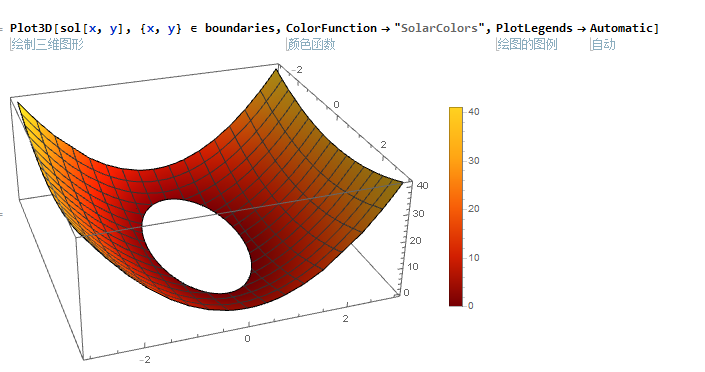#### 精确解的图像

``````Plot3D[
Piecewise[{{x^2 + 4*y^2 - 4, x^2 + 4 y^2 > 4}, {None, x^2 + 4 y^2 <= 4}}],
{x, -3, 3}, {y, -3, 3},
PlotRange -> {{-3, 3}, {-3, 3}, {0, 40}}, BoxRatios -> {1, 1, 1}]``````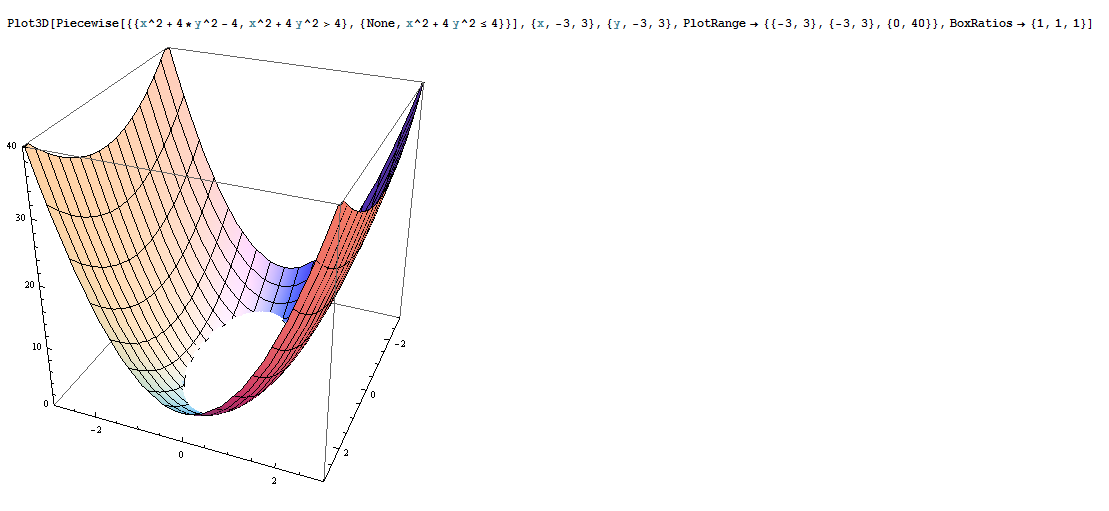### 参考链接

http://reference.wolfram.com/language/tutorial/NDSolvePDE.html.zh：偏微分方程的数值解

• 微信公众号
• 关注微信公众号
•• QQ群
• 我们的QQ群号
•• 本文由 发表于 2017年10月5日12:02:56
• 转载请务必保留本文链接：https://mathpretty.com/8272.html